#《程序设计解题策略》——1.2　利用最小生成树及其扩展形式解题

0
0
01. 云栖社区>
2. 博客>
3. 正文

## 《程序设计解题策略》——1.2　利用最小生成树及其扩展形式解题

### 1.2　利用最小生成树及其扩展形式解题

1) 构建最小生成树。
2) 应用最小生成树原理优化算法。

1) 最优比率生成树。
2) 最小k度限制生成树。
3) 次小生成树的解法。
1.2.1　最小生成树的思想和应用

A是一棵最小生成树的子集，如果边(u，v)不属于A且A∪{(u，v)}仍然是某一棵最小生成树的子集，就称(u，v)为集合A的安全边。由此引出计算最小生成树的一般思想：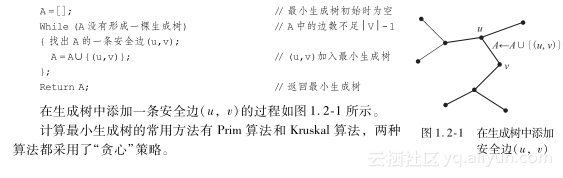（1） Prim算法https://yqfile.alicdn.com/b81e9720703be5615cf549bcfb89b5ceaf162e42.png" >

Void PRIM(G,w,r)　　　　　　　　// 图G的相邻矩阵为w，构造以r为根的最小生成树
{
Q=V［G］;// 所有节点送入队列Q
for（每个u∈Q）key［u］=∞// 所有节点的最短距离值初始化为∞
key［r］=0;// 根r的最短距离值为0
π［r］=NIL;// r的父节点为空,即r进入生成树
while(Q<>Ф）// 若队列不空
{ u=EXTRACT-MIN(Q);// 队列Q中距离值最小的节点u出队进入生成树
// 边权小于原距离值，则v的父亲调整为u，距离值调整为（u,v）的边权
if(v∈Q)&&(w(u,v)<key［v］){π［v］=u;key［v］=w(u,v) };
}
}


（2） Kruskal算法https://yqfile.alicdn.com/697aab8769321576a4addb111baff4561acf04aa.png" >

Void KRUSKAL(G,w)
{A=Ф　　　　　　　　　　　　　　　// 最小生成树的子集初始化为空
For(每个节点v∈V［G］)v自成一个集合；

for（顺序搜索E中的每条边(u,v)）
{ if （u和v属于两个不同的集合）
{ A=A∪{(u,v);// (u,v)进入生成树A

}
}
return A;// 返回生成树A
}


【1.2.1　Arctic Network】
【问题描述】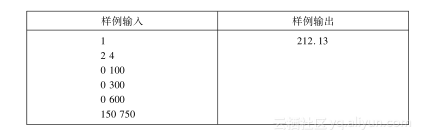1) 正向思考：在已知连通分支数S的基础上求连通分支内边长的上限D。
2) 逆向思维：在已知连通分支内边长上限D的基础上求连通分支数S。

【定理1.2-1】　如果去掉所有权值大于D的边后，最小生成树被分割成为S个连通分支，图也被分割成为S个连通分支。

#include <iostream>
#include <cstring>
#include <cstdio>
#include <cmath>
#include <algorithm>
using namespace std ;

const int MAXN = 505 ;

struct　Edge　　　　　　　　　　　　　　　// 边的结构定义
{
int　start , end ;// 边的两个端点
double length ;// 边长
bool visit ;// 最小生成树的边标志
} edge［MAXN * MAXN］ ;// 边集

struct　Point// 点的结构定义
{
int　x , y ;// 坐标
} point［MAXN］ ;// 点集

int　father［MAXN］,Count ;// 节点i所在连通分支的代表节点为father［i］，完全图的
// 边数为Count

double　getlength( Point a , Point b )// 计算a点和b点的欧氏距离
{
double len ;
len=sqrt(double((a.x-b.x)*(a.x-b.x)+(a.y-b.y)*(a.y-b.y ))) ;
return len ;
}

bool　cmp( Edge a , Edge b )// 比较a和b的边长大小
{
return a.length < b.length ;
}

int　find( int x )// 计算x点所在连通支的代表节点
{
if( father［x］ == x ) return x ;
father［x］ = find( father［x］ ) ;
return father［x］　　　　　　　;
}

bool Union( int x , int y )// 合并：若x和y不在同一连通分支，则代表节点序号小的
// 连通分支并入代表节点序号大的连通分支，并返回合并标志
{
int　f1 = find( x ) ;// 计算x所在连通分支的代表节点f1
int　f2 = find( y ) ;// 计算y所在连通分支的代表节点f2
if( f1 == f2 ) return false ;// 若x和y在同一连通分支，则返回false
else if( f1<f2 )father［f1］=f2;// 合并x和y所在的连通分支，返回合并标志
else father［f2］ = f1 ;
return true ;
}

double kruskal( int n )// 使用Kruskal算法计算最小生成树
{
int　i , j = 0 ;
double sum = 0 ;
for(i=0;i< n;i ++) father［i］=i;// 初始时每个节点自成一个连通分支
sort( edge,edge+Count,cmp ) ;// Count条边按边长递增顺序排列
for(i=0;i<Count && j<n;i++)// 枚举每条边，构造n-1条边的最小生成树
{
if (Union(edge［i］.start,edge［i］.end))// 若第i条边的两个端点不在同一连通分支，则加入最小生成树
{
sum += edge［i］.length;// 边长计入最小生成树的边权和
edge［i］.visit　= 1 ;// 第i条边为最小生成树的树边
j ++ ;
}
}
return sum ;// 返回最小生成树的边权和
}

int　main()
{
int　T , S , P ;// 测试用例数T，卫星频道数S，哨所数P
int　i , j ;
scanf( "%d" , & T ) ;// 输入测试用例数
while( T -- )// 依次处理每个测试用例
{
Count=0 ;// 完全图的边数初始化
scanf( "%d%d",&S ,&P );// 输入卫星频道数和哨所数
for( i=0;i<P;i++ )// 输入每个哨所的坐标
scanf( "%d%d" , & point［i］.x , & point［i］.y ) ;
for( i=0;i<P-1;i++ )// 以哨所为节点构造完全图
for( j=i+1;j<P;j++)
{// 记下第Count条边的端点和边长
edge［Count］.start=i;edge［Count］.end=j;
edge［Count］.length=getlength( point［i］ , point［j］ ) ;
edge［Count］.visit=0 ;// 设置非生成树边标志
Count ++ ;// 完全图的边数+1
}
kruskal( P ) ;// 构造完全图的最小生成树
for( i=Count-1;i>= 0;i-- ){// 按照边长递减顺序枚举最小生成树的S条树边，第S大的树边
// 即为连接网络的最小D值
if( edge［i］.visit )
{
S -- ;
if( S == 0 ) break ;
}
}
printf( "%.2f＼n",edge［i］.length );// 输出连接网络的最小D值
}
return 0 ;
}

【1.2.2　Robot】
【问题描述】https://yqfile.alicdn.com/b8247c07d2a305af923be4f37c1f60e75f99ebcd.png" >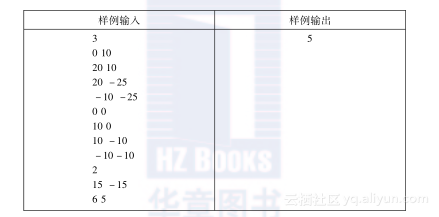https://yqfile.alicdn.com/f9e0a1797f99d450a42fc69c78648405a7afa90d.png" >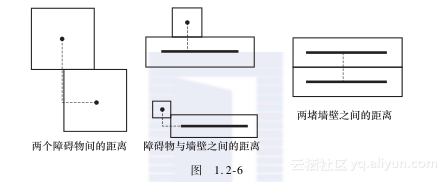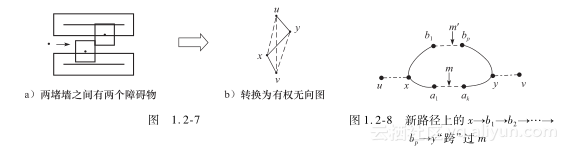#include <cstdio>
#include <cstring>
#include <cmath>
#include <iostream>
using namespace std ;
typedef struct {　　　　　　　　　　// 坐标定义
int x,y;
} pt;

int m,n;// 直线墙数m，障碍物数n
pt up［32］,dn［32］;// 第i个转弯点的“上部分”墙体坐标up［i］、
// “下部分”墙体坐标dn［i］
pt pr［128］;// 障碍物坐标

int min(int a,int b){// 计算和返回整数a、b的较小者
if (a<b) { return a; } else { return b; }
}

int max(int a,int b){// 计算和返回整数a、b的较大者
if (a>b) { return a; } else { return b; }
}

int ISIN(int a,int b,int c){// 返回区间［b,c］含a的标志
if ((a>=b) && (a<=c)) { return 1; } else { return 0; }
}

int gr［128］［128］;// 连通图的相邻矩阵
int wel［128］;// 节点的距离值
int inv［128］;// 节点在生成树的标志
int clc［128］;// 经过当前转弯处的障碍物标志

int dst(int x1,int y1,int x2,int y2,int x3,int y3){
// 当前线段(x1,y1)-(x2,y2), 障碍物(x3,y3)，计算障碍物与线段
// 在X或Y轴向上的最大距离
int d;
if (y1==y2){// 若线段是水平的,则返回max{ 线段与障碍物的垂直距离, X轴向上
// 障碍物与线段的最近距离∣障碍物的x坐标在线段的x区间外}
d =abs(y3-y1);
if (ISIN(x3,min(x1,x2),max(x1,x2))==0)
{ d=max(d, min(abs(x3-x1),abs(x3-x2)) ); }
} else {// 若线段是垂直的（x1=x2），则返回max{ 线段与障碍物的水平距离,
//  Y轴向上障碍物与线段的最近距离∣障碍物的y坐标在线段的x区间外}
d = abs(x3-x1);
if (ISIN(y3,min(y1,y2),max(y1,y2))==0)
{ d=max(d, min(abs(y3-y1),abs(y3-y2)) ); }
}
return d;
}

int pass(int x1,int y1,int x2,int y2,int h0,int h){
// h转弯处线段h和线段h+1的上下两部分的X区间为［x1,x2］，
// Y区间为［y1,y2］，h的奇偶标志位h0。往连通图添边

int i,j,v,v1;
for (i=0;i<n;i++){// 标志经过h转弯处的障碍物
if ((ISIN(pr［i］.x,x1,x2)) && (ISIN(pr［i］.y,y1,y2))){
clc［i］=1;
}　else { clc［i］=0; }
}
for (j=0;j<n;j++){// 枚举经过h转弯处的一对障碍物j和i，计算(j，i)的边权
if (clc［j］==0) { continue; }
for (i=j+1;i<n;i++){
if (clc［i］==0) { continue; }
v =max(abs(pr［i］.x-pr［j］.x),abs(pr［i］.y-pr［j］.y));
gr［i］［j］=v;
gr［j］［i］=v;
}
}
for (i=0;i<n;i++){// 枚举经过h转弯处的障碍物i，调整(n,i)和(n+1,i)的边权
if (clc［i］==0) { continue; }
v = dst( up［h］.x , up［h］.y , up［h+1］.x , up［h+1］.y , pr［i］.x , pr［i］.y );
v1 = dst( dn［h］.x , dn［h］.y , dn［h+1］.x , dn［h+1］.y , pr［i］.x , pr［i］.y );
gr［n］［i］ = min(gr［n］［i］,v);
gr［i］［n］ = min(gr［i］［n］,v);
gr［n+1］［i］ = min(gr［n+1］［i］,v1);
gr［i］［n+1］ = min(gr［i］［n+1］,v1);
}
if (h0==0) {// 若h为偶数，则调整（n,n+1）的边权
v = y2-y1;
} else{ v=x2-x1; }
gr［n］［n+1］ = min(v,gr［n］［n+1］);
gr［n+1］［n］ = min(v,gr［n+1］［n］);
return 0;
}

int deik(void){// 使用Prim算法计算连通图gr［］［］的最小生成树
int c,i,v,v1;
c = n+2;// 计算最小生成树的节点数
for (i=0;i<c;i++){// 所有节点在生成树外，距离值为∞
wel［i］=0x7FFF;
inv［i］=0;
}
wel［c-2］ = 0;// 节点n为根，其距离值为0
for (;;){
v1 = 0x7fff; v=-1;// 在生成树外寻找距离值最小(v1)的节点v
for (i=0;i<c;i++)
if (inv［i］==0)
if (wel［i］<v1) { v1=wel［i］; v=i; }
if (v<0) { break; }// 若生成树外无节点，则成功退出；否则v进入生成树
inv［v］ = 1;
for (i=0;i<c;i++)// 对生成树外节点的距离值进行松弛操作
if ((inv［i］==0) && (gr［v］［i］<0x7fff))
wel［i］ = min( max(v1,gr［v］［i］) , wel［i］ );
}
return wel［c-1］;// 返回最小生成树的最长边
}

int main(void){
int test;
scanf("%d",&test);// 输入测试用例数
while(test--){// 依次处理每个测试用例
int i,a,b,c,d,r;
scanf("%d ",&m);// 输入直线墙的线段数
// 输入m+1个转弯点（包括端点）的“上部分”墙体坐标和下部分”
// 墙体坐标
for (i=0;i<=m;i++) scanf("%d %d ",&up［i］.x,&up［i］.y);
for (i=0;i<=m;i++) scanf("%d %d ",&dn［i］.x,&dn［i］.y);
scanf("%d ",&n);// 输入障碍物数和每个障碍物的坐标
for (i=0;i<n;i++) scanf("%d %d ",&pr［i］.x,&pr［i］.y);
memset(gr,0x11,128*128*sizeof(int));
for (i=0;i<m;i++){// 枚举每个转弯点。计算邻接于第i个转弯点的两条线段的X轴区间
// ［a,c］、Y轴区间［b,d］，根据该信息往连通图添边
a = min( min( up［i］.x , up［i+1］.x ) , min( dn［i］.x , dn［i+1］.x ) );
b = min( min( up［i］.y , up［i+1］.y ) , min( dn［i］.y , dn［i+1］.y ) );
c = max( max( up［i］.x , up［i+1］.x ) , max( dn［i］.x , dn［i+1］.x ) );
d = max( max( up［i］.y , up［i+1］.y ) , max( dn［i］.y , dn［i+1］.y ) );
pass(a,b,c,d,i%2,i);
}
r = deik();// 使用Prim算法计算和输出连通图的最小生成树的最长边
printf("%d＼n",r);
}
return 0;
}


【1.2.3　Qin Shi Huangs National Road System】
【问题描述】#include <cstdio>
#include <cstring>
#include <iostream>
#include <cmath>

using namespace std;

const int X = 1002;　　　　　　　　　　　　// 城市数的上限
#define INF 1e12// 无穷大的定义

struct node// 城市的结构定义
{
int x,y,w;// 坐标(x,y)、人口w
}p［X］;// 城市序列

int n;// 城市数
double map［X］［X］;// 完全图的相邻矩阵
double dis［X］,total,ans;// 节点距离值dis［］，最小生成树的边权和total，最大比率
// A/B为ans
bool use［X］;// 生成树的节点标志或当前集合标志
int pre［X］;// 生成树则节点i的父指针为pre［i］

double dist(int x1,int y1,int x2,int y2)// (x1,y1)和(x2,y2)间的欧氏距离
{
return sqrt((x1-x2)*(x1-x2)+(y1-y2)*(y1-y2));
}

void prim()// 使用Prim算法求完全图map［］［］的最小生成树
{
memset(pre,0,sizeof(pre));// 前驱初始化
total = 0;// 最小生成树的边权和初始化
memset(use,false,sizeof(use));// 所有节点未在生成树
for(int i=1;i<=n;i++)dis［i］=INF;// 所有节点的距离值初始化
dis［1］ = 0;// 节点1为根，距离值为0
int k;
double MIN;
for(int i=0;i<n;i++)// 寻找生成树外距离值最小的节点k（距离值为MIN）
{
MIN = INF;
for(int j=1;j<=n;j++)
if(!use［j］&&dis［j］<MIN)MIN = dis［k = j］;
use［k］=true;total+=MIN;// k进入最小生成树，距离值计入边权和
for(int j=1;j<=n;j++)// 对生成树外节点的距离值进行松弛操作
if(!use［j］&&dis［j］>map［k］［j］)dis［j］=map［k］［j］,pre［j］=k;
}
}

void dfs(int i)// 通过深搜i的后趋点找到所在集合use［］
{
use［i］=true;// i进入集合
for(int j=1;j<=n;j++)// 递归i后驱点中未被访问的节点
if(!use［j］&&pre［j］==i)dfs(j);
}

void solve()// 计算和输出最大比率A/B
{
double temp;
ans = 0;
int a,b;
for(int i=1;i<=n;i++)// 枚举每条边
{
if(pre［i］==0)continue;// 若i为根，则继续枚举
temp=total-map［i］［pre［i］］;// 删除边（i,pre［i］）
memset(use,false,sizeof(use));// 计算i所在的集合use［］
dfs(i);
a = b = 0;
for(int j=1;j<=n;j++)// 在删除边（i,pre［i］）后得到的两个点集中分别找最大价值的点
{
if(use［j］&&j!=pre［i］)// j为pre［i］所在集合的点，调整该集合最大价值
a=max(a,p［j］.w);
else if(!use［j］&&j!=i)// j为i所在集合的点，调整该集合最大价值
b=max(b,p［j］.w);
}
ans=max(ans,(a+b)/temp);// 调整ans
}
printf("%.2lf＼n",ans);// 输出最大比率A/B
}

int main()
{
freopen("sum.in","r",stdin);// 输入文件读准备
freopen("sum.out","w",stdout);// 输出文件写准备
int t;cin>>t;// 输入测试用例数
while(t--)
{
scanf("%d",&n);// 输入城市数
for(int i=1;i<=n;i++)// 输入每个城市的坐标和人口
{
scanf("%d%d%d",&p［i］.x,&p［i］.y,&p［i］.w);
for(int j=i;j>=1;j--)// 构造完全图的相邻矩阵map［］［］
map［i］［j］ = map［j］［i］ = dist(p［i］.x,p［i］.y,p［j］.x,p［j］.y);
}
prim();// 计算完全图的最小生成树
solve();// 计算和输出最大比率A/B
}
return 0;
}

1.2.2　最优比率生成树的思想和应用

【性质1.2-1】　z单调递减。

【性质1.2-2】　z(max(l))=0。

Prerat和rate初始化；
while(true)
{

rate=sumc/sums；
if (rate-prerate≤精度要求) break;// 不可能再优化，成功退出
prerate=rate;// 记下当前生成树的比率
}



【1.2.4　Desert King】
【问题描述】https://yqfile.alicdn.com/a022b63b4b00575e69cc7171e0e6e6fb91af4418.png" >

方法1：二分法#include<iostream>
#include<algorithm>
#include<cstring>
#include<cmath>
using namespace std;
#define MAX 1001　　　　　　　　　　　　　　　// 节点数的上限
#define INF 1000000000// 定义无穷大
struct node// 节点的结构定义
{
double x,y,h;// 坐标和高度
}dot［MAX］;// 节点序列

inline double dis(double x1,double y1,double x2,double y2)
// （x1,y1）至(x2,y2)的欧氏距离
{
return sqrt( (x2-x1)*(x2-x1)+(y2-y1)*(y2-y1) );
}
double graph［MAX］［MAX］;// 相邻矩阵
inline void creat(int n,double l)// 构造新图的相邻矩阵graph［］［］
{
int i,j;
for(i=1;i<=n;i++)
{
for(j=1;j<=n;j++)
{
graph［i］［j］=fabs(dot［i］.h-dot［j］.h)-l*dis(dot［i］.x,dot［i］.y,dot［j］.x,dot［j］.y);
}
}
}
inline double prim(double graph［MAX］［MAX］,int n)
// 使用Prim算法构造连通图graph［］［］的最小生成树
{
bool visit［MAX］={0};// 所有节点在生成树外
int mark;// 进入生成树的节点序号
double dis［MAX］;// 节点的距离值
double ans=0;// 最小生成树的边权和初始化
int i,j;
visit［1］=true;// 以节点1为根，计算各节点的距离值
for(i=1;i<=n;i++)
dis［i］=graph［1］［i］;
for(i=1;i<n;i++)// 逐条边加入生成树
{
int minnum=INF;// 在生成树外的节点中寻找距离值最小的节点mark，
// 其距离值为minnum
for(j=1;j<=n;j++)
{
if(!visit［j］&&dis［j］<=minnum)
{
minnum=dis［j］;
mark=j;
}
}
visit［mark］=true;　ans+=dis［mark］;
// mark进入生成树，距离值计入边权和
for(j=1;j<=n;j++)// 对生成树外节点的距离值进行松弛操作
{
if(!visit［j］&&graph［mark］［j］<dis［j］)
dis［j］=graph［mark］［j］;
}

}
return ans;// 返回最小生成树的边权和
}

int main()
{
int i,j;
int n;// 节点数
double res;// 当前生成树的边权和
while(scanf("%d",&n))// 反复输入节点数，直至输入0为止
{
if(n==0)
break;
for(i=1;i<=n;i++)// 输入每个节点的坐标和高度
{
scanf("%lf%lf%lf",&dot［i］.x,&dot［i］.y,&dot［i］.h);
}
double front,rear;　front=0;　rear=100;
// 通水渠道总成本/总长度的比值区间初始化
double mid;// 区间中间指针
double pre=0.0;
while(front<=rear)// 若未找到最小比值，则循环
{
mid=(front+rear)/2;// 计算中间指针
creat(n,mid);// 构造新图的相邻矩阵graph［］［］
res=prim(graph,n);// 计算新图的最小生成树的边权和
if(fabs(res-pre)<=0.0005)// 若边权和为0，则退出
break;
else if(res>0.0005)// 若边权和>0，则在右区间寻找；否则在左区间寻找
front=mid;
else
rear=mid;
}
printf("%.3lf＼n",mid);// 输出通水渠道总成本/总长度的最小比
}
return 0;
}方法2：迭代法#include<iostream>
#include<algorithm>
#include<cstring>
#include<cmath>
using namespace std;
#define MAX 1001// 节点数的上限
#define INF 1000000000// 无穷大
struct node// 节点的结构定义
{
double x,y,h;// 位置（x,y），高度h
}dot［MAX］;// 节点序列

inline double dis(double x1,double y1,double x2,double y2)
// 计算线段(x1,y1)-(x2,y2)的欧氏距离
{
return sqrt( (x2-x1)*(x2-x1)+(y2-y1)*(y2-y1) );
}

double graph［MAX］［MAX］;// 图的相邻矩阵
double c［MAX］［MAX］;// 存储节点间高度差的绝对值
double s［MAX］［MAX］;// 存储节点间的欧氏距离

inline void creatcs(int n)// 计算每对村庄间的距离和海拔高度差的绝对值
{
int i,j;
for(i=1;i<=n;i++)
{
for(j=1;j<=n;j++)
{
c［i］［j］=fabs(dot［i］.h-dot［j］.h);
s［i］［j］=dis(dot［i］.x,dot［i］.y,dot［j］.x,dot［j］.y);
}
}
}

inline void creat(int n,double l)// 用c［i］［j］-l*s［i］［j］重新构图
{
int i,j;
for(i=1;i<=n;i++)
{
for(j=1;j<=n;j++){ graph［i］［j］=c［i］［j］-l*s［i］［j］;}
}
}

double sumc;// 最小生成树边长1（两端点高度差的绝对值）的和
double sums;// 最小生成树边长2（两端点的欧氏距离）的和

inline void prim(double graph［MAX］［MAX］,int n)// 使用Prim算法计算图的最小生成树
{
sumc=0;sums=0;// 最小生成树边长1的和与边长2的和初始化
bool visit［MAX］={0};// 初始时所有节点在生成树外
int mark;// 生成树外节点中距离值最小的节点
int pre［MAX］;// 节点父指针
double dis［MAX］;// 节点距离值（与生成树节点的最短边长）
int i,j;
visit［1］=true;// 节点1进入生成树，计算所有节点的距离值和父指针
for(i=1;i<=n;i++){ dis［i］=graph［1］［i］;pre［i］=1;}
for(i=1;i<n;i++)// 逐条边添入生成树
{
int minnum=INF;// 计算生成树外节点中距离值最小（minnum）的节点mark
for(j=1;j<=n;j++)
{
if(!visit［j］&&dis［j］<=minnum){ minnum=dis［j］;mark=j;}
}
visit［mark］=true;// 节点mark进入生成树，与生成树相连边的高度差的绝对值
// 计入sumc，欧氏距离计入sums
sumc+=c［pre［mark］］［mark］;sums+=s［pre［mark］］［mark］;
for(j=1;j<=n;j++)// 调整生成树外与mark相邻节点的距离值
{
if(!visit［j］&&graph［mark］［j］<dis［j］){dis［j］=graph［mark］［j］;pre［j］=mark;}
}
}
}

int main()
{
int i,j;
int n;// 村庄数
while(scanf("%d",&n))// 反复输入村庄数，直至输入0
{
if(n==0) break;
for(i=1;i<=n;i++)// 输入每个村庄的位置和海拔高度
{ scanf("%lf%lf%lf",&dot［i］.x,&dot［i］.y,&dot［i］.h); }
creatcs(n);// 计算每对村庄间的距离和海拔高度差的绝对值
double prerate=30.0;double rate=30.0;// 比率的最小值和最大值初始化
while(true)
{
creat(n,rate);// 用c［i］［j］-rate*s［i］［j］重新构图graph［］［］
prim(graph,n);// 计算图的最小生成树
rate=sumc/sums;// 计算当前生成树的比率
if(fabs(rate-prerate)<0.001)break;// 若比率相同于上次生成树的比率，则成功退出
prerate=rate;// 记下当前生成树的比率
}
printf("%.3lf＼n",rate);// 输出通水渠道总成本与总长度的最小比值
}
return 0;
}


1.2.3　最小k度限制生成树的思想和应用

【引理1.2-1】　设T′、T是图G的两棵不同的生成树，

E(T′)＼E(T)={a1，a2，…，an}
E(T)＼E(T′)={b1，b2，…，bn}


【定理1.2-2】　设T是G的k度限制生成树，则T是G的最小k度限制生成树当且仅当下面三个条件同时成立：
1) 对于G中任何两条与v0关联的边所产生的T的可行交换都是不可改进的。
2) 对于G中任何两条与v0不关联的边所产生的T的可行交换都是不可改进的。
3) 对于T的任何两个可行交换(+a1，-b1)和(+a2，-b2)，若a1、b2与v0关联，b1、a2不与v0关联，则有ω(b1)+ω(b2)≤ω(a1)+ω(a2)。

E(T′)＼E(T)={a1，a2，…，an}
E(T)＼E(T′)={b1，b2，…，bn}


【定理1.2-3】　设T是G的最小k度限制生成树，E0是G中与v0有关联的边的集合，E1=E0＼E(T)，E2=E(T)＼E0，A={(+a，-b)a∈E1，b∈E2}，设ω(a′)-ω(b′)=min{ω(a)-ω(b)(+a，-b)∈A}，则T+a′-b′是G的一个最小k+1度限制生成树。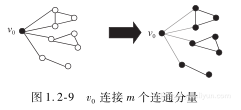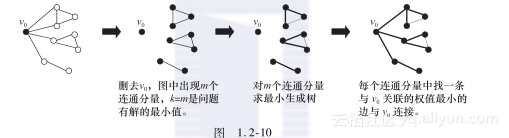https://yqfile.alicdn.com/5437cb85622d5ed29b01a57a2d5d55baa99064a1.png" >

【1.2.5　Picnic Planning】
【问题描述】
Contortion Brothers是一群著名的马戏团小丑，众所周知，他们有着令人难以置信的能力，能把无限多的自己塞进即使是最小的车辆。在演出淡季，他们喜欢聚在当地的一个公园举行年度的柔术演员会议(Annual Contortionists Meeting，ACM)。然而，他们不仅受限于狭窄的空间，而且缺钱，所以他们尝试找一个方法使得参加会议的每个人的车的里程数最小(以节省汽油、减少磨损等)。为此，他们要把自己挤塞进尽可能少的汽车内，并且尽量使他们的所有车辆的总里程数最小化。这就导致了这样的情况，许多人开车到一个同伴的家，把他们的车放在那里，与同伴挤进一辆车中。然而，在公园也有一条约束：在野餐地点的停车场只能容纳有限数量的汽车，所以必须考虑到整体的计算。此外，由于公园的门票原因，一旦一个同伴的车到了公园，他就不能放下他的乘客，然后离开去接其他的同伴。现在请你编写一个程序来解决他们的里程数最小化问题。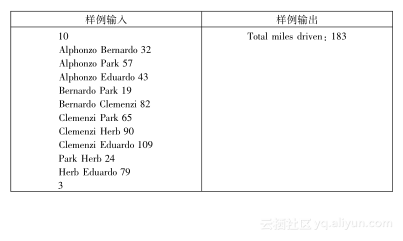1) 将v0从图中删除，将得到p个连通分量。
2) 对每个连通分量求最小生成树。
3) 从每个连通分量中找出与v0关联的权值最小的边，与v0相连接，这样将得到v0的最小p度生成树。
4) 如果k

5) 如果k≥p，则考虑构建p+1度最小生成树，即加入每一条与v0相连且不在当前生成树的边。
6) 显然，在步骤5)添加一条与v0相连的树外边，必然会存在一个环，删掉该环中与v0不关联的权值最大边，将得到一棵最小生成树，且v0是p+1度的。若p+1度最小生成树的边权和大于p度最小生成树的边权和，则直接输出p度最小生成树的边权和；否则重复步骤5)、6)，直至k度最小生成树出现为止。

#include<iostream>
#include<cstdio>
#include<cstring>
#include<algorithm>
#include<cmath>
#include<queue>
#include<stack>
#include<string>
#include<vector>
#include<cstdlib>
#include<map>
#include<set>
using namespace std;
#define inf 0x3f3f3f3f// 定义无穷大
const int maxn = 25;// 节点数的上限
int m,n,k,g［maxn］［maxn］,dis［maxn］,pre［maxn］,ans;
// 图的节点数n，边数m，相邻矩阵g［］［］；节点i的距离值（生成树外的节点i连接生成树内节点的最短边长）
// 为dis［i］，该节点的前驱为pre［i］；k度最小生成树的边权和为ans
// 标志vis［］
struct node// 边节点的结构定义
{
int u,v,len;// 边(u，v)的权为len
node(){}
node(int u, int v, int len):u(u),v(v),len(len){}
}del［maxn］;// del［i］保存节点0到节点i的路径上的最长边
int kk;

void prim(int st)// 使用Prim算法求包含st的连通块的生成树
{
vis［st］ = 1;// st进入生成树
memset(pre, -1, sizeof(pre));// 所有节点的前驱指针初始化为空
for (int i = 1; i < n; ++i)// 定义所有节点的距离值和前驱指针
{ dis［i］ = g［st］［i］; pre［i］ = st; }
while(1)
{
int tmp = inf, nt = st;// 在树外节点中找出距离值最小的节点nt
for (int i = 1; i < n; ++i)
{
if (!vis［i］ && dis［i］ < tmp) { nt = i; tmp = dis［i］; }
}
if (st == nt) break;// 该连通分量的最小生成树已形成
if(g［0］［nt］<g［0］［kk］)kk=nt;// kk保存该连通分量到节点0距离最近的点
// 将边加入生成树中
vis［nt］=1; ans+=dis［nt］;// nt为生成树内的节点，其距离值累计入生成树的总权和
for (int i=1; i<n; ++i)// 调整生成树外每个节点的前驱和距离值
{
if(!vis［i］&&dis［i］>g［nt］［i］)// 若i在树外且(nt,i)的边长小于i的距离值，则调整i
// 的前驱和距离值
{ pre［i］ = nt; dis［i］ = g［nt］［i］; }
}
}
}

void dfs(int cur,int cpre,int u,int v)// 修改当前连通分量中到达节点0的路径上的最大边。
// cur为当前节点，cpre为当前节点的前驱，(u,v)表示
// 调整前路径上的最大边
{
for (int i = 1; i < n; ++i)// 枚举树中除(cpre,cur)外与cur相连的每条边
{
if (cpre != i && link［cur］［i］)
{
if(cpre==-1||g［cur］［i］>=g［u］［v］)
// 若当前边权大于之前保存的最大边，则当前边记为最大
// 边，继续递归；否则最大边不变，继续递归
{ del［i］=node(cur, i, g［cur］［i］); dfs(i, cur, cur, i); }
else { del［i］ = node(u, v, g［u］［v］); dfs(i, cur, u, v); }
}
}
}

void solve()// 计算和输出k 度最小生成树的边权和
{
for (int i = 1; i < n; ++i)// 枚举节点0外不在生成树的每个节点i
{
if (vis［i］) continue;
k--; kk = i;// 计算剩余的度数，记下i
prim(i);// 计算以i为根的最小生成树
// (i,0)加入生成树，累计边权
dfs(kk, -1, -1, -1);// 调整当前连通分量的最长边
}
while(k--)
{
int c = 0, nt = 0;
for (int j = 1; j < n; ++j)// 枚举生成树外邻接节点0的所有节点
{
if (link［0］［j］ || g［0］［j］ == inf) continue;
if(c < del［j］.len - g［0］［j］)// 找出使得边权和减少量最大的添删操作，即添边（0,nt），
// 删除节点0到节点nt的路径上的最长边后使得边权和
// 减少量最大
{ nt=j; c=del［j］.len-g［0］［j］;// 记下边权和的减少量
}
}
if (c == 0) break;// 若生成树的边权和无法减少，则退出循环
ans -= c;// 调整生成树的边权和
// 删除最长边
dfs(nt, 0, -1, -1);// 调整当前连通分量的最长边
}
printf("Total miles driven: %d＼n",ans);// 输出k 度最小生成树的边权和
}

void init()// 输入边信息，构造图的相邻矩阵
{
char s1［20］,s2［20］;
int w, u, v;
n = 0;// 节点数初始化
map <string,int> name;
map <string,int>::iterator it1,it2;
name.clear();
name［"Park"］ = n++;// 根节点定义为0
memset(g, 0x3f, sizeof(g));// 图的相邻矩阵初始化为空
memset(vis,0,sizeof(vis));// 初始时所有节点在生成树外，生成树的相邻矩阵为空
for (int i = 0; i < m; ++i)
{
scanf("%s %s %d", &s1, &s2, &w);// 输入边信息
it1 = name.find(s1); it2 = name.find(s2);
if (it1 != name.end()) u = it1->second;
// 定义两个端点的节点编号
else { name［s1］ = n; u = n++; }
if (it2 != name.end()) v = it2->second;
else { name［s2］ = n; v = n++; }
if (g［u］［v］ > w) g［u］［v］ = g［v］［u］ = w;
// 往相邻矩阵添边
}
scanf("%d", &k);// 输入度的限制数
ans = 0;// k 度最小生成树的边权和初始化
}

int main()
{
while(scanf("%d", &m) != EOF)// 反复输入边数
{
init();// 输入边信息，构造图的相邻矩阵
solve();// 计算和输出k 度最小生成树的边权和
}
return 0;
}


1.2.4　次小生成树的思想和应用

【定理1.2-4】　设T是图G的最小生成树，如果T1满足ω(T1)=min{ω(T′)T′∈N(T)}，则T1是G的次小生成树。

E(T′)＼E(T)={a1，a2，…，at}，
E(T)＼E(T′)={b1，b2，…，bt}，其中t≥2。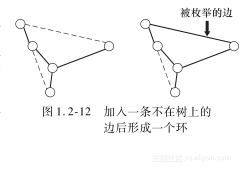1) 求图G的最小生成树T(时间复杂度为O(Vlog2V+E))；
2) 做一步预处理，通过简单的宽度优先搜索求出树上每两个节点之间的路径上的权值最大的边(时间复杂度为O(V2))；
3) 枚举图中不在树上的边(时间复杂度为O(E))。有了刚才的预处理，我们就可以用O(1)的时间得到环上权值最大的边。

【1.2.6　次小生成树Tree】
【问题描述】https://yqfile.alicdn.com/2895849814dde1c189bf75d0dce0d1ad6cdce9b4.png" >

#include <iostream>
#include <vector>
#include <string.h>
#include <string>
#include <stdio.h>
#include <cstdio>
#include <algorithm>
using namespace std;

#define REP(I, N) for (int I=0;I<int(N);I++)
#define SZ(A) int(A.size())// 定义容器大小
#define PB push_back// 在vector尾部加入一个数据
#define MP(A, B) make_pair(A, B)// 将A和B两个值视为一个单元

typedef long long LL;
typedef double DB;

typedef pair<int, int> PII;
typedef vector<PII> VII;// 最小生成树邻接表的元素类型，第一个整数为父节点
// 序号，第二个整数为通往父亲的边序号

template<class T> inline void checkMin(T &a, T b){if (b<a) a=b;};
// 取最小值

struct edge {// 边表元素的结构类型
int a , b , w ;// (a,b)的权为w
int id ;// 边序号
friend bool operator<( edge x , edge y ){return x.w<y.w;}
// 边按权值递增排序
} ;

const　LL INF = 1ll<<60 ;// 定义∞
const　int N = 100000 , M = 300000 ;// 定义节点数和边数的上限

edge　 E［M］ ;// 边表
int　　P［N］ , R［N］ ;// x节点所在并查集的代表节点为p［x］，秩为R［x］
bool　 InMst［M］ ;// 该边在生成树的标志

VII　　adj［N］ ;// 最小生成树的邻接表
int　　Fa［N］ , Fb［N］ , vis［N］ ;// 最小生成树中i节点的父节点为Fa［i］，父边序号为
// Fb［i］,通往根的路径序号为vis［i］
int w［M］,s［M］ ,_i ;// 边i的权w［i］，删除树边i后应添加的树外边权s［i］，
// 路径序号_i
int　　n , m , q ;// 节点数n，边数m
LL　　 mst ;// 最小生成树的边权和

void　 Make(int x) { P［x］ = x ; }// 建立仅含x节点的并查集
int　Find(int x){ if(P［x］!= x)P［x］=Find(P［x］);return P［x］;}
// 返回x所在并查集的代表节点

void　 Union(int x, int y)// 合并x和y所在的并查集
{ if (R［x］<R［y］)P［y］=x;
else { if (R［x］ == R［y］)R［y］++ ; P［x］ = y ; }
}

void　 Kruskal()// 使用Kruskal 算法计算最小生成树
{
sort(E, E+m) ;// 按照权值递增的顺序排列边
REP(i, n) Make(i) ;// 为每个节点建立并查集
REP(i, m)// 顺序枚举每条边
{
if(Find(E［i］.a)==Find(E［i］.b))continue;
// 若第i条边在同一并查集中，则继续枚举边；否则将该边
// 加入最小生成树
Union( Find(E［i］.a) , Find(E［i］.b) ) ;
PB( MP(E［i］.a, E［i］.id) ) ;
mst+=E［i］.w ;// 该边权计入最小生成树的边权和
}
}

void　 dfs(int u, int p)// 建立生成树的父子关系
{
{
int v = adj［u］［i］.first ;
if (v != p) Fa［v］=u,Fb［v］=adj［u］［i］.second, dfs(v, u);
}
}

int　 lca(int a, int b)// 计算生成树中a和b的最近公共祖先
{
while(a!=0){ vis［a］=_i;a=Fa［a］;}// 将a至根路径上每个节点的路径序号设为_i
vis［0］ = _i ;
while(vis［b］!= _i)b = Fa［b］;// 从b出发向上寻找第1个路径序号为_i的节点b
return b ;// 返回最近公共祖先b
}

void link(int a,int b,int c)// 边权为c的树外边(a,b)，将树内连接a和b的路径上
// 边的s值设为c
{
int p=lca(a, b), t ;// 计算树中a和b的最近公共祖先p
#define Cloze(a)if(!s［a］ && c!=w［a］)s［a］=c
// 将边a的s值设为c
// 分别将树中a和b通往公共祖先p的两条路径上途径边的s值设为c
while (a!=p){ Cloze(Fb［a］);t=Fa［a］,Fa［a］=p ,a=t;}
while (b!=p){ Cloze(Fb［b］);t=Fa［b］,Fa［b］=p,b=t;}
}

void　 Stat()// 计算删除树内边后应添加的树外边权s［］
{
dfs(0, -1) ;// 在以节点0为根的生成树中，建立每个节点的父关系
REP(i, m)// 枚举不在生成树的每条边，将树内连接两端点的路径上
// 节点的s值设为该边权
{
if(InMst［E［i］.id］) continue ;
_i=i+1;// 通往根的路径序号为_i（用于计算边i的两端点在树内
// 的公共祖先）
}
}

int　　main()
{
scanf( "%d%d" , &n , &m ) ;// 读节点数和边数
// 读入每条边的信息,构造相邻矩阵
REP(i, m) scanf( "%d%d%d", &E［i］.a,&E［i］.b,&E［i］.w ),E［i］.a--,E［i］.b--, E［i］.id =i,
w［i］=E［i］.w ;
Kruskal() ;// 使用Kruskal算法求最小生成树
Stat() ;// 计算删除树内边后应添加的树外边权s［］
LL ans = INF ;// 次小生成树的边权和初始化
REP(i, m)// 枚举生成树的边，若删除该边并加入树外边后可使边权和
// 增量最小，则调整次小生成树的边权和
if ( InMst［i］ && s［i］ )checkMin(ans, mst-w［i］+s［i］) ;
printf( "%lld＼n" , ans );// 输出次小生成树的边权和
}


【1.2.7　ACM contest and Blackout】
【问题描述】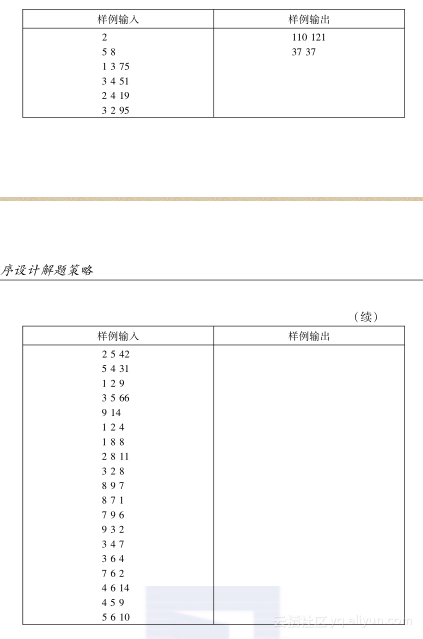#include<cstdio>
#include<cstring>
#define INF 1<<30　　　　　　　　　　　// 定义无穷大
int map［110］［110］,n,m;// 图的相邻矩阵map［］［］，节点数n，边数m
int vis［110］,use［110］［110］,low［110］;// 节点i的树内标志vis［i］，距离值low［i］，
// use［i］［j］=0i和j无边
1(i,j)最小生成树T
2(i,j)∈最小生成树T
int pre［110］,F［110］［110］;// 节点i的前驱pre［i］，生成树中i至j路径上的最大
// 边权为F［i］［j］
int max(int x,int y)// 求x和y的较大者
{
return x>y?x:y;
}
int prim(int s,int t)// 使用Prim算法计算以s节点为根的最小生成树
{
int ans=0;// 最小生成树的边权和初始化
memset(pre,-1,sizeof(pre));// 每个节点的父指针初始化为空
pre［s］ = -1;// s的父指针为-1
memset(F,0,sizeof(F));// 生成树中路径的最大边初始化
for(int i = 1; i <= n; i++)// 初始时所有节点在生成树外，距离值为∞
{
vis［i］ = 0;low［i］ = INF;
}
low［1］ = 0;// 节点1的距离值为0
for(int i = 1; i <= n; i++)// 逐个节点加入生成树
{
int temp = INF,tp=-1;// 寻找生成树外距离值最小的节点tp（距离值temp）
for(int j=1;j<=n; j++)if(!vis［j］&&temp>low［j］){ tp = j;temp=low［j］;}
if(tp==-1) continue;
int k=tp;　int v=pre［k］;
if(v!=-1)// 若k非根，则（v, k）进入生成树
{
use［k］［v］=use［v］［k］=2;
for(int j=1;j<=n;j++)// 搜索生成树内的每个节点j，调整F［j］［k］
if(vis［j］)F［j］［k］=max(F［j］［v］,map［v］［k］);
}
vis［k］=1; ans+=low［k］;// k进入最小生成树，距离值累计入边权和
for(int j=1;j<=n; j++)// 调整生成树外的每个节点的距离值和父指针
if(!vis［j］&&low［j］>map［k］［j］){ low［j］ = map［k］［j］;pre［j］ = k; }
}
return ans;// 返回最小生成树的权值和
}

int second_mst(int x)// 根据最小生成树的权值和x计算次小生成树的权值和
{
int res=x,ans=INF;// 记下最小生成树的权值和，次小生成树的权值和初始化
for(int i=1;i<=n;i++)// 搜索生成树外每条满足条件的边(i,j)（i与j间在生成树内存
// 在路径）：若加入(i,j)、删除i与j间路径上的最长边可使权值和的增量最小，则调整次小生成树的权值和
for(int j = 1; j <= n; j++)
if(use［i］［j］==1 && map［i］［j］!=INF && F［i］［j］!=INF)
{
if(res+map［i］［j］-F［i］［j］<ans)ans=res+map［i］［j］-F［i］［j］;
}
return ans;// 返回次小生成树的权值和
}

int main()
{
int t;
scanf("%d",&t);// 输入测试用例数
while(t--)
{
scanf("%d%d",&n,&m);// 输入节点数和边数
for(int i = 0; i<=n; i++)// 相邻矩阵map初始化
for(int j = 0; j<=n; j++) map［i］［j］ = INF;
memset(use,0,sizeof(use));// 初始时所有节点间的无边
int x,y,z;
for(int i=0; i<m; i++)// 输入m条边的信息，构造相邻矩阵并设这些边在树外
{
scanf("%d%d%d",&x,&y,&z);
map［x］［y］=map［y］［x］=z;
use［x］［y］=use［y］［x］=1;
}
int ans=prim(1,n);// 使用Prim算法计算最小生成树的边权和
int ans1=second_mst(ans);// 计算次小生成树的边权和
printf("%d %d＼n",ans,ans1);// 输出最小生成树的边权和与次小生成树的边权和
}
return 0;
}

+ 关注